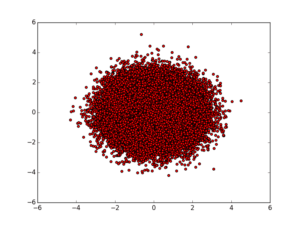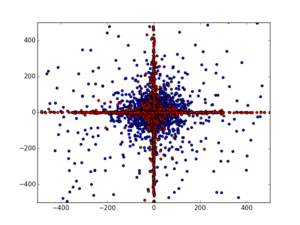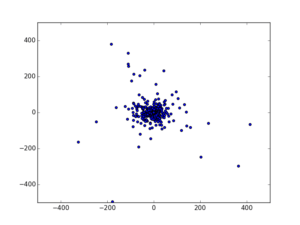# When the tail is bigger than the dogMost people (at least those with college education) are well aware of how exponential growth works. The typical (correct) intuition is that when things are growing exponentially, they may initially look like nothing, in fact things may go very slow for quite a while, but eventually there is an explosion and exponential growth eventually outpaces everything sub-exponential. What is less commonly appreciated is that exponential decay works similarly - things exist, get smaller and effectively at some point become nonexistent. It is almost as if there was a discrete transition. Let us keep that in mind while we discuss some probability theory below.

#### Gauss and Cauchy

Gauss and Cauchy were two very famous mathematicians, both having countless contributions in various areas of mathematics. Coincidentally, two seemingly similarly looking probability distributions are named after these two individuals. And although many people working in data science and engineering have relatively good understanding of Gaussian distribution (otherwise known as "normal" distribution), Cauchy distribution is less known. It is also a very interesting beast, as it is an example of a much less "normal" distribution than Gaussian and most intuitions from typical statistics fail in the context of Cauchy. Although Cauchy like distributions are not in the focus of mainstream statistics education, they are extremely important and describe many natural phenomena, as we shall discuss by the end of this post. Let us look at the distribution densities:

The left is the so called bell curve (Gaussian distribution with expected value$\mu=0$ and variance$\sigma^2=1$) defined as:$f(x)=\frac{1}{\sqrt{2\pi\sigma^2}}e^{-\frac{(x-\mu)}{\sigma^2}}$

while the one on the right is defined as:$g(x)=\frac{1}{\pi(1+x^2)}$

which is sometimes referred to as the standard Cauchy distribution. They both look very similar, hence many people would expect these probability distributions to have similar properties. They do differ in one important way though: Gaussian distribution decays exponentially while Cauchy does not. As it turns out this makes a colossal difference.

#### Long tail beast

I will be visualizing the properties of samples from these distributions as 2 dimensional scatter plots for convenience. Just as I attempt to do that comes the first surprise: when we sample two independent samples from a Gaussian distribution and plot them on a scatter plot as independent dimensions we get a circularly symmetric object:

import numpy as np
import matplotlib.pyplot as plt
N=100000

G1_x = np.random.randn(N)
G1_y = np.random.randn(N)

plt.scatter(G1_x,G1_y, marker='o', c='r')
plt.show()In fact we can get the same (in terms of distribution) cloud of points if we sample a length of a vector from the origin from a Gaussian distribution and then sample uniformly a random angle between$[0, 2\pi]$.

import numpy as np
import matplotlib.pyplot as plt
N=100000

G = np.random.randn(N)
al = np.random.rand(N)
G_x = G*np.sin(np.pi*2*al)
G_y = G*np.cos(np.pi*2*al)

plt.scatter(G_x,G_y, marker='o', c='b')
plt.show()Although the exact realizations of those processes will obviously yield different scatter plots, in terms of distribution they are the same! It may seem obvious but it by no means is, Gaussian distributions co-vary exactly in a way to make it possible. Obviously it is not the case for uniform distribution on a finite segment, but it is also not true for other bell shaped distributions as we may find out by running the following code:

import numpy as np
import matplotlib.pyplot as plt
N=100000

C = np.random.standard_cauchy(N)
al = np.random.rand(N)
C_x = C*np.sin(np.pi*2*al)
C_y = C*np.cos(np.pi*2*al)

C1_x = np.random.standard_cauchy(N)
C1_y = np.random.standard_cauchy(N)

plt.scatter(C_x,C_y, marker='o', c='b')
plt.scatter(C1_x,C1_y, marker='o', c='r')
plt.xlim([-500,500])
plt.ylim([-500,500])
plt.show()As we see (red) the two independent samples of Cauchy distribution plotted on a scatter plot as independent dimensions vary too much to create a circular shape and instead create this cross like structure. It is as if this variability was imbalanced between the center and the tail of the distribution: things are either in the center or in the tail, but a coincidence of both samples in the tail it too rare. This in fact is because this distribution has a non negligible tail. The blue scatter plot (that of circularized distribution) at first sight resembles that of the Gaussian, but as we will see in a moment they are quite different.

We could plot both distributions on the same plot, but we note that circularized Cauchy distribution appears to vary in a broad range of values (-500, 500) whereas the Gaussian is essentially limited to (-4, 4). To fit them together I will scale Cauchy distribution by a factor of 100.

import numpy as np
import matplotlib.pyplot as plt
N=100000

G = np.random.randn(N)
G1_x = np.random.randn(N)
G1_y = np.random.randn(N)

plt.scatter(G1_x,G1_y, marker='o', c='r')

C = 0.01*np.random.standard_cauchy(N)
al = np.random.rand(N)
C_x = C*np.sin(np.pi*2*al)
C_y = C*np.cos(np.pi*2*al)

plt.scatter(C_x,C_y, marker='o', c='b')
plt.ylim([-5,5])
plt.xlim([-5,5])
plt.show()Now we see clearly, that circularized Cauchy appears to have a sharper spike in the center, but there are also more speckles away from the center (which is the tail). In fact of we zoom out 10x from this image (by modifying xlim and xlim by 10x) we get:The entire Gaussian sample is contained in the small region next to the center of the image, where Cauchy appears to have speckles very far. We can repeat this experiment by zooming out another 10x and increasing the sample size to N=1000000. For clarity I'll plot them on separate plots:Gaussian distribution is completely restricted to the small region above, while Cauchy:actually has samples all over the place. This in essence is the exponential decay of the Gaussian visualized: anything beyond several standard deviations has so negligible probability that becomes effectively non-existent. So even though in theory Gaussian distribution is non-zero everywhere, practically it has a support of several standard deviations. In contrast Cauchy actually has a long tail and the events well away from the mode (the most frequent event) are actually quite possible.

#### Strange properties

Although by looking at the distribution curves we can quickly estimate which values will be sampled most frequently (the peak of the distribution), in the case of the Gaussian that value aligns exactly with the mean of the distribution which is defined as the integral over all possible values of the random variable weighted by the probability of that value. In the finite sample case the mean is just the sum of all the samples divided by the number of samples. Although this may be surprising, in the case of Cauchy distribution such defined integral does not converge! In essence Cauchy distribution does not have a mean! Of course we can always take the sum of the samples and divide it by the number of samples, but in the case of Cauchy distribution such estimator will jump all over the place and never converge to anything fixed. This is quite important and may be counterintuitive: the random process of sampling from Cauchy distribution varies so much, that one cannot average it out!

Not only that, the variance which measures the amount of "random spread" in the case of the Gaussian is infinite for Cauchy! In fact Cauchy does not have any higher moments! In some ways, Cauchy is a lot more wild and random than Gauss.

#### Domesticated randomness

Gaussian distribution symbolizes a certain kind of domesticated randomness. The fast decay of the tail of that distribution allow it to have mean and variance and of the distributions with those properties defined it is in some sense the most general (e.g. has the highest entropy). In the world of such domesticated random variables, Gaussian distribution plays a central role as a population limit in what is called "central limit theorem". In principle it says that the sum of random variables (which are independent and all have finite mean and variance) will converge (in terms of distribution) to a Gaussian distribution. This is why the Gaussian distribution is so often found in nature: in all cases where the observable is a result of averaging of many "non-wild" random events.

#### Wild randomness

Cauchy distribution on the other hand represents a completely different world of "wild randomness". In this world tails don't decay exponentially, and this causes a whole lot of trouble. Central limit theorem does not work, laws of large numbers don't work, many many things we learn in statistics classes don't work. Is such randomness found in nature as well? Indeed it is: so called power laws being a prominent example of long tail distributions. In fact, there are numerous places where long tail (Cauchy like) distributions appear: Zipf law for languages, critical phase transitions, lifespans of species, frequency of earthquakes and avalanches, distribution of sizes of celestial bodies, distribution of sizes of supernovae, stock market variability etc.

In many ways the wild randomness is more fundamental than the domesticated one. Think of a casino, a well defined system with limits where randomness is extremely domesticated (to bring profit to casino owners and entertainment to the guests). But the casino itself could be torn down by an earthquake (a wild random event), and although much stuff in it is a domesticated randomness, that domesticated randomness is  there only because of the limits generated by that particular highly organized structure. Once that structure is gone, the limits may no longer hold and the randomness may get wild. In almost all cases of physical phenomena that obey Gaussian randomness, we could think of an outside physical event that will destroy that constrained environment. In that sense the Universe is wild, but there are temporary pockets  of "domesticated randomness" in it, where the Gaussian goodies are available.

#### Summary

What we learn in statistics classes is heavily centered on the Gaussian distribution, primarily because it is domesticated and we know how to handle random processes in that narrow domain. There is however a fallacy among many people working in statistics and data science that all randomness is like that and effectively any random process in nature is more or less Gaussian. This could not be further from the truth. We don't learn much about wild long tail randomness in statistics classes only because there are way fever practical results and we understand those regimes much less, not because they are not present in nature.

My personal hunch is that intelligence is somehow related to the ability to deal with "wild randomness". My working hypothesis is that tail events are really the most important for survival (both in the sense of being most dangerous as well as offering the best opportunities). Ability to detect and deal with these tails in all cases aligns with intelligent behavior. That being said, practically all our contemporary approaches to AI are in many ways conceptually restricted to domesticated randomness...

If you found an error, highlight it and press Shift + Enter or click here to inform us.